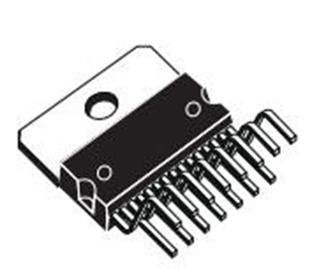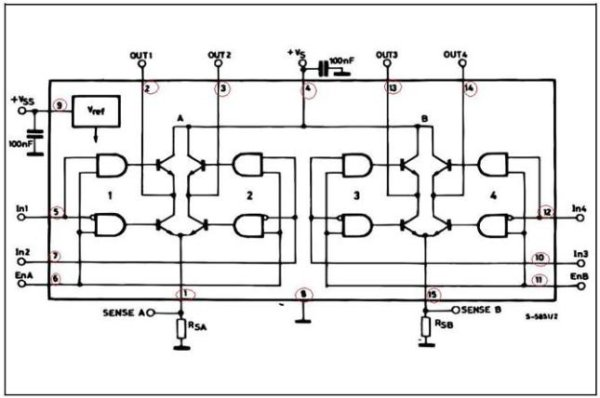# IC-Based H-Bridge Tutorial

An alternative to using a transistorized H-Bridge circuit is the L298 Dual Full-Bridge Driver. It is very easy to control and it doesn’t require any calculation. Simply knowing your motor’s required current and the IC’s current capacity is enough. For a price though.In order to learn this, you need to first download the IC’s datasheet file. Simply google “L298 Datasheet” or click the link —> L298 Datasheet.H-bridge circuits are used to control the direction of the rotation of the motor. The L298 is a dual H-Bridge circuit. It is composed of 2 H-Bridges, therefore a maximum of 2 motors can be controlled simultaneously. The amazing part of this component is that the control voltage reads logic level voltages, no need to compute for series resistors – you will have to simply connect the pin of the microcontroller (or any logic level devices) to the corresponding control pin of the L298 directly.

Given Example:

In this tutorial, we will use the L298 for driving and controlling a 1A DC Motor, with Vcc of 12V applied, and controlled by a PIC Microcontroller which I will be using for my steering wheels on my line follower robot.

Step 1: Begin with knowing the IC’s maximum ratings.

From the L298 datasheet shows the absolute max ratings.

The maximum voltage applied to the motor to be used (as well as the IC) is 50V. And the logic high input on the control voltage is 7V.The Io or Output Current is one of the important factors that need to be considered. It is stated when you use a DC Motor, the maximum allowed current is 2 amps. If your motor requires a much larger current (for example 2.5Amps) then you might want to find other h-bridge devices.

READ  Laser show for poor man using PIC18F1220 microcontroller

In this case, the motor that we are using is rated 1A DC, Vs = 12V, and logic high supply from a microcontroller is 5V.

For more detail: IC-Based H-Bridge Tutorial